# Methods of Charging of a body

The different methods of Charging of a body are :-

(a) friction, (b) conduction, (c) induction, (d) thermionic ionization or thermionic emission (e) photoelectric effect and (f) field emission.

(a) Charging by Friction :
When a neutral body is rubbed against other neutral body then some electrons are transferred from one body to other. The body which can hold electrons tightly, draws some electrons and the body which can not hold electrons tightly, looses some electrons. The body which draws electrons becomes negatively charged and the body which looses electrons becomes positively charged.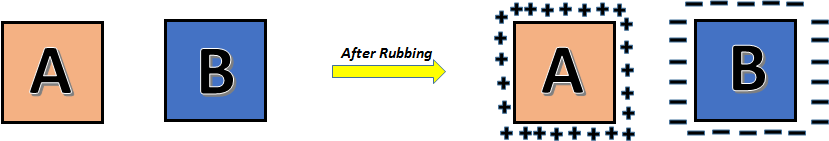For example :

Suppose a glass rod is rubbed with a silk cloth. As the silk can hold electrons more tightly and a glass rod can hold electrons less tightly (due to their chemical properties), some electrons will leave the glass rod and gets transferred to the silk. So in the glass rod their will be deficiency of electrons, therefore it will become positively charged. And in the silk there will be some extra electrons, so it will become negatively charged.

(b) Charging by conduction :

There are three types of material in nature:-

(i) Conductor : Conductors are the material in which the outer most electrons are very loosely bounded, so they are free to move (flow). So in a conductors, there are large number of free electrons.
Ex. Metals like Cu, Ag, Fe, Al………….

(ii) Insulator or Dielectric or Nonconductor : Non-conductors are the materials in which outer most electrons are very tightly bounded, so they cannot move (flow). Hence in a non-conductor there is no free electrons. Ex. plastic, rubber, wood etc.
(iii) Semi conductor : Semiconductor are the materials which have free electrons but very less in number.

Now lets see how the charging is done by conduction.

In this method we take a charged conductor ‘A’ and an uncharged conductor ‘B’. When both are connected some charge will flow from the charged body to the uncharged body. If both the conductors are identical & kept at large distance, if connected to each other, then charge will be divided equally in both the conductors otherwise they will flow till their electric potential becomes same.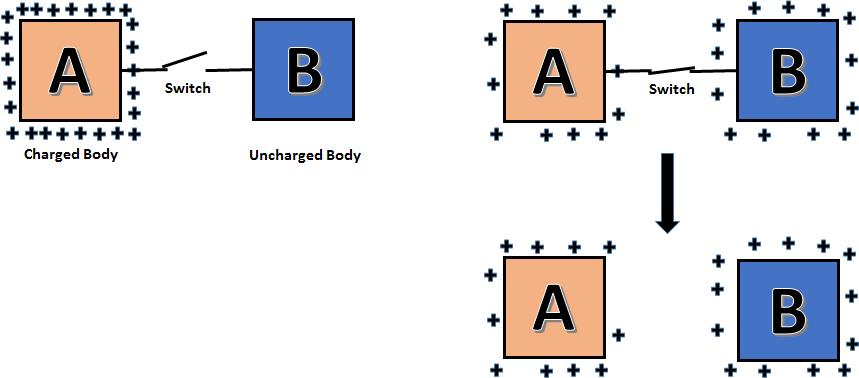(c) Charging by Induction : To understand this, lets have introduction to induction.We have studied that there are lot of free electrons in the conductors. When a charge particle +Q is brought near a neutral conductor. Due to attraction of +Q charge, many electrons (–ve charges) come closer and accumulate on the closer surface.

On the other hand a positive charge (deficiency of electrons) appears on the other surface. The flow of charge continues till there is resultant force on free electrons of the conductor becomes zero. This phenomena is called induction, and charges produced are called induced charges.
A body can be charged by induction in the following two ways :

Method I :

Step 1. Take an isolated neutral conductor.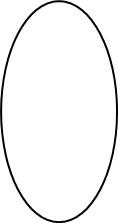Step 2. Bring a charged rod near to it. Due to the charged rod, charges will induce on the conductor.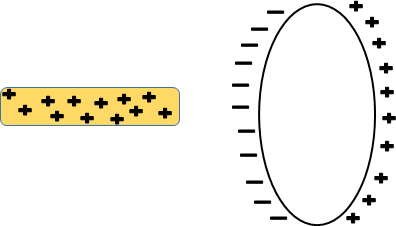Step 3. Connect another neutral conductor with it. Due to attraction of the rod, some free electrons will move from the right conductor to the left conductor and due to deficiency of electrons positive charges will appear on right conductor and on the left conductor there will be excess of electrons due to transfer from right conductor.Step 4. Now disconnect the connecting wire and remove the rod.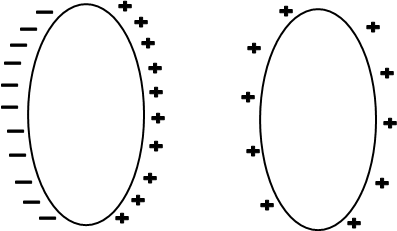The first conductor will be negatively charged and the second conductor will be positively charged.

The first conductor will be negatively charged and the second conductor will be positively charged.

Method II:

Step 1. Take an isolated neutral conductor.Step 2. Bring a charged rod near to it. Due to the charged rod, charges will induce on the conductor.Step 3. Connect the conductor to the earth (this process is called grounding or earthling). Due to attraction of the rod, some free electrons will move from earth to the conductor, so in the conductor there will be excess of electrons due to transfer from the earth, so net charge on conductor will be negative.Step 4. Now disconnect the connecting wire. Conductor becomes negatively charge.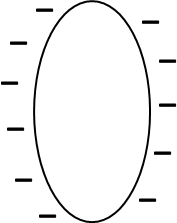(d) Thermionic emission : When the metal is heated at a high temperature then some electrons of metals are ejected and the metal becomes positively charged.(e) Photoelectric effect : When light of sufficiently high frequency is incident on metal surface then some electrons gains energy from light and come out of the metal surface and remaining metal becomes positively charged.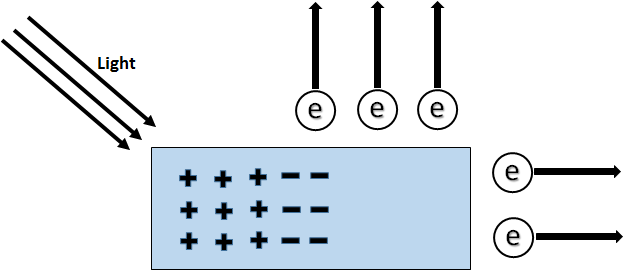(f) Field emission : When electric field of large magnitude is applied near the metal surface then some electrons come out from the metal surface and hence the metal gets positively charged.To watch video lecture of the above topic click below:-